# Presentation 1st

4 de Nov de 2014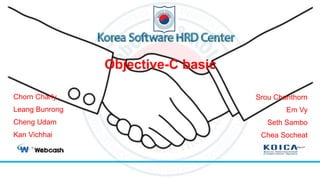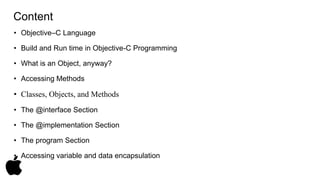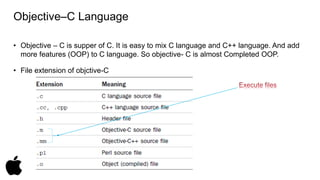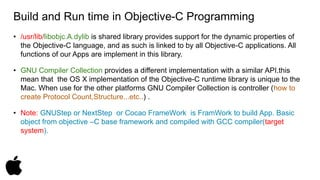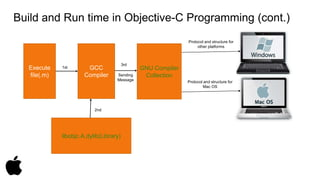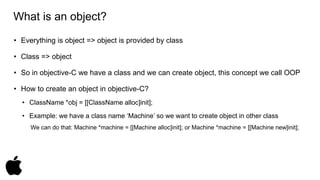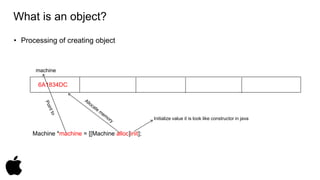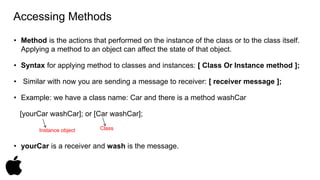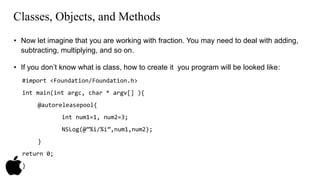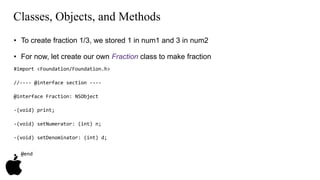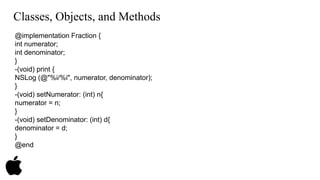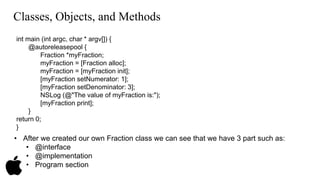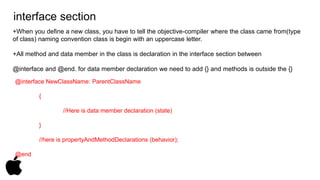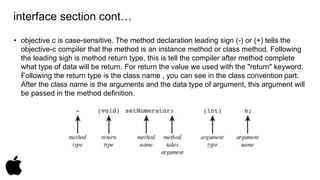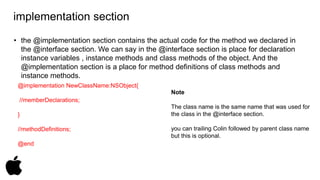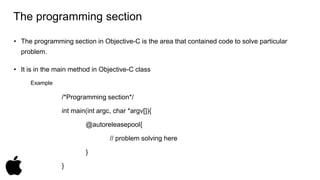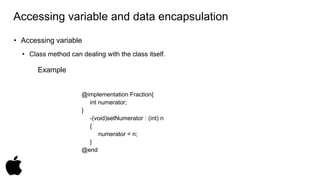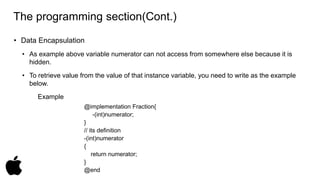1 de 19

### Presentation 1st

• 1. Objective-C basic Chorn Charly Leang Bunrong Cheng Udam Kan Vichhai Srou Chanthorn Em Vy Seth Sambo Chea Socheat
• 2. Content • Objective–C Language • Build and Run time in Objective-C Programming • What is an Object, anyway? • Accessing Methods • Classes, Objects, and Methods • The @interface Section • The @implementation Section • The program Section • Accessing variable and data encapsulation
• 3. Objective–C Language • Objective – C is supper of C. It is easy to mix C language and C++ language. And add more features (OOP) to C language. So objective- C is almost Completed OOP. • File extension of objctive-C
• 4. Build and Run time in Objective-C Programming • /usr/lib/libobjc.A.dylib is shared library provides support for the dynamic properties of the Objective-C language, and as such is linked to by all Objective-C applications. All functions of our Apps are implement in this library. • GNU Compiler Collection provides a different implementation with a similar API.this mean that the OS X implementation of the Objective-C runtime library is unique to the Mac. When use for the other platforms GNU Compiler Collection is controller (how to create Protocol Count,Structure...etc..) . • Note: GNUStep or NextStep or Cocao FrameWork is FramWork to build App. Basic object from objective –C base framework and compiled with GCC compiler(target system).
• 5. Build and Run time in Objective-C Programming (cont.) 1st Sending Message Protocol and structure for other platforms Protocol and structure for Mac OS Execute file(.m) GCC Compiler GNU Compiler Collection 2nd libobjc.A.dylib(Library) 3rd
• 6. What is an object? • Everything is object => object is provided by class • Class => object • So in objective-C we have a class and we can create object, this concept we call OOP • How to create an object in objective-C? • ClassName *obj = [[ClassName alloc]init]; • Example: we have a class name ‘Machine’ so we want to create object in other class We can do that: Machine *machine = [[Machine alloc]init]; or Machine *machine = [[Machine new]init];
• 7. What is an object? • Processing of creating object machine 6A1834DC Machine *machine = [[Machine alloc]init]; Initialize value it is look like constructor in java
• 8. Accessing Methods • Method is the actions that performed on the instance of the class or to the class itself. Applying a method to an object can affect the state of that object. • Syntax for applying method to classes and instances: [ Class Or Instance method ]; • Similar with now you are sending a message to receiver: [ receiver message ]; • Example: we have a class name: Car and there is a method washCar [yourCar washCar]; or [Car washCar]; Instance object Class • yourCar is a receiver and wash is the message.
• 9. Classes, Objects, and Methods • Now let imagine that you are working with fraction. You may need to deal with adding, subtracting, multiplying, and so on. • If you don’t know what is class, how to create it you program will be looked like: #import <Foundation/Foundation.h> int main(int argc, char * argv[] ){ @autoreleasepool{ int num1=1, num2=3; NSLog(@”%i/%i”,num1,num2); } return 0; }
• 10. Classes, Objects, and Methods • To create fraction 1/3, we stored 1 in num1 and 3 in num2 • For now, let create our own Fraction class to make fraction #import <Foundation/Foundation.h> //---- @interface section ---- @interface Fraction: NSObject -(void) print; -(void) setNumerator: (int) n; -(void) setDenominator: (int) d; • @end
• 11. Classes, Objects, and Methods @implementation Fraction { int numerator; int denominator; } -(void) print { NSLog (@"%i/%i", numerator, denominator); } -(void) setNumerator: (int) n{ numerator = n; } -(void) setDenominator: (int) d{ denominator = d; } @end
• 12. Classes, Objects, and Methods int main (int argc, char * argv[]) { @autoreleasepool { Fraction *myFraction; myFraction = [Fraction alloc]; myFraction = [myFraction init]; [myFraction setNumerator: 1]; [myFraction setDenominator: 3]; NSLog (@"The value of myFraction is:"); [myFraction print]; } return 0; } • After we created our own Fraction class we can see that we have 3 part such as: • @interface • @implementation • Program section
• 13. interface section +When you define a new class, you have to tell the objective-compiler where the class came from(type of class) naming convention class is begin with an uppercase letter. +All method and data member in the class is declaration in the interface section between @interface and @end. for data member declaration we need to add {} and methods is outside the {} @interface NewClassName: ParentClassName { //Here is data member declaration (state) } //here is propertyAndMethodDeclarations (behavior); @end
• 14. interface section cont… • objective c is case-sensitive. The method declaration leading sign (-) or (+) tells the objective-c compiler that the method is an instance method or class method. Following the leading sigh is method return type, this is tell the compiler after method complete what type of data will be return. For return the value we used with the "return" keyword. Following the return type is the class name , you can see in the class convention part. After the class name is the arguments and the data type of argument, this argument will be passed in the method definition.
• 15. implementation section • the @implementation section contains the actual code for the method we declared in the @interface section. We can say in the @interface section is place for declaration instance variables , instance methods and class methods of the object. And the @implementation section is a place for method definitions of class methods and instance methods. @implementation NewClassName:NSObject{ //memberDeclarations; } //methodDefinitions; @end Note The class name is the same name that was used for the class in the @interface section. you can trailing Colin followed by parent class name but this is optional.
• 16. The programming section • The programming section in Objective-C is the area that contained code to solve particular problem. • It is in the main method in Objective-C class Example /*Programming section*/ int main(int argc, char *argv[]){ @autoreleasepool{ // problem solving here } }
• 17. Accessing variable and data encapsulation • Accessing variable • Class method can dealing with the class itself. Example @implementation Fraction{ int numerator; } -(void)setNumerator : (int) n { numerator = n; } @end
• 18. The programming section(Cont.) • Data Encapsulation • As example above variable numerator can not access from somewhere else because it is hidden. • To retrieve value from the value of that instance variable, you need to write as the example below. Example @implementation Fraction{ -(int)numerator; } // its definition -(int)numerator { return numerator; } @end